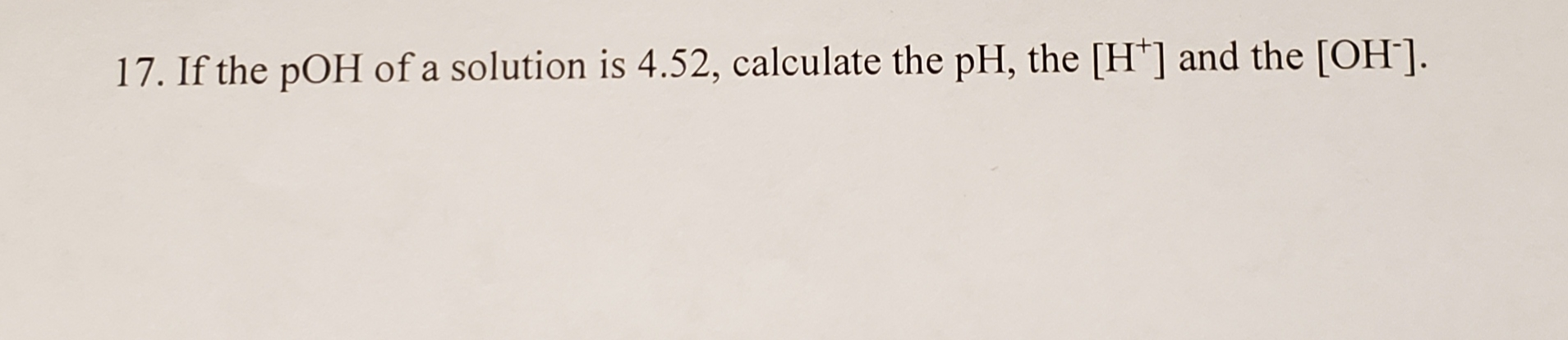# 17. If the pOH of a solution is 4.52, calculate the pH, the [H'] and the [OH].

Question
115 viewshelp_outlineImage Transcriptionclose17. If the pOH of a solution is 4.52, calculate the pH, the [H'] and the [OH]. fullscreen
check_circle

Step 1

Determine the pH of the given solution from pOH using the below formula.

Step 2

The hydronium ion concentration, [H+] can be calculated from the above obtained pH as follows,

Step 3

The hydroxide ion concentration, [OH-] can be...

### Want to see the full answer?

See Solution

#### Want to see this answer and more?

Solutions are written by subject experts who are available 24/7. Questions are typically answered within 1 hour.*

See Solution
*Response times may vary by subject and question.
Tagged in

### General Chemistry